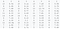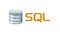# 1. Anscombe Quartet

Anscombe Quartet was developed by Francis Anscombe, a Statistician, in 1973. The Anscombe’s quartet comprises four datasets that have nearly identical statistical properties, yet appear very different when graphed.Anscombe’s Quartet Dataset

When we find the mean of all the X’s in the above dataset, they are approximately 9 and the mean of all the Y’s are approximately 7.5. Also the Standard Deviation of all the X’s are approximately 3.16 and the Standard Deviation of all the Y’s are 1.94 …

# Selecting, Counting & Filtering in SQLSource : dev.to

SQL (Structured Query Language) is a language used for extracting and organizing data stored in a relational database.

There are 5 Commands in SQL and they are:

• DDL(Data Definition Language).
• DML(Data Manipulation Language).
• DQL(Data Query Language).
• DCL(Data Control Language).
• TCL(Transaction Control Language).

# Linear Regression in Machine Learning

Concept:

Linear Regression is a supervised Machine Learning algorithm used to make predictions on a continuous quantity whose behavior is linear in nature. For instance, Mrs Johnson wants to predict the price of a shoes, certain factors can affect the price of shoes, it could be the material used to make it, where it is made, the color and the fashion. Linear Regression can help with this prediction.

## The equation of line is given by : y = m x + b

If a data is linear, then a line of best fit can be drawn for that data. Linear Regression follows from the equation of line from our basic algebra given by: y=mx+c…## Margaret Awojide

Statistician | Mathematician | Data Scientist